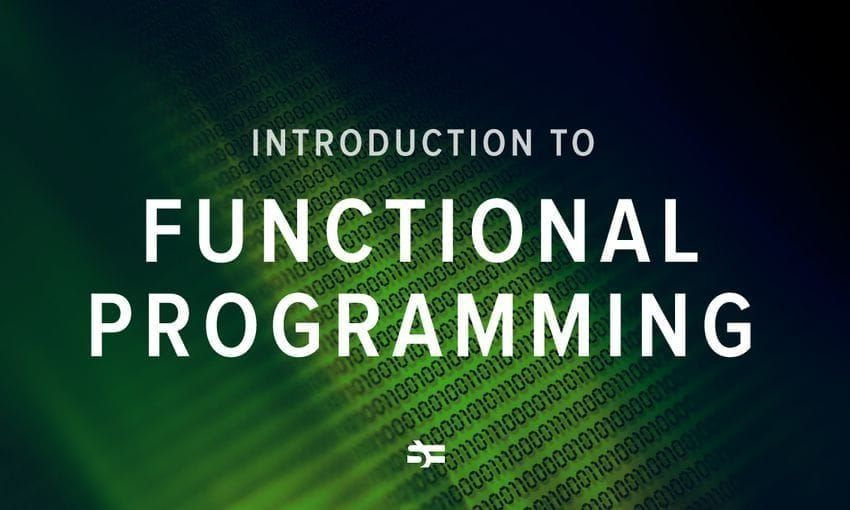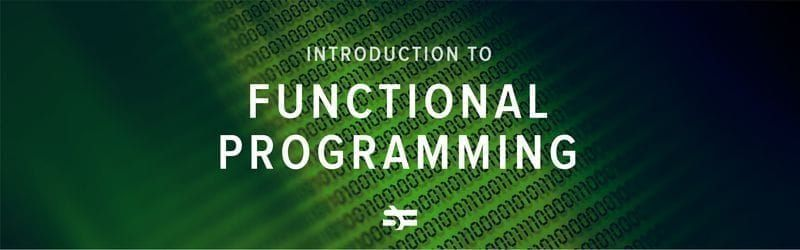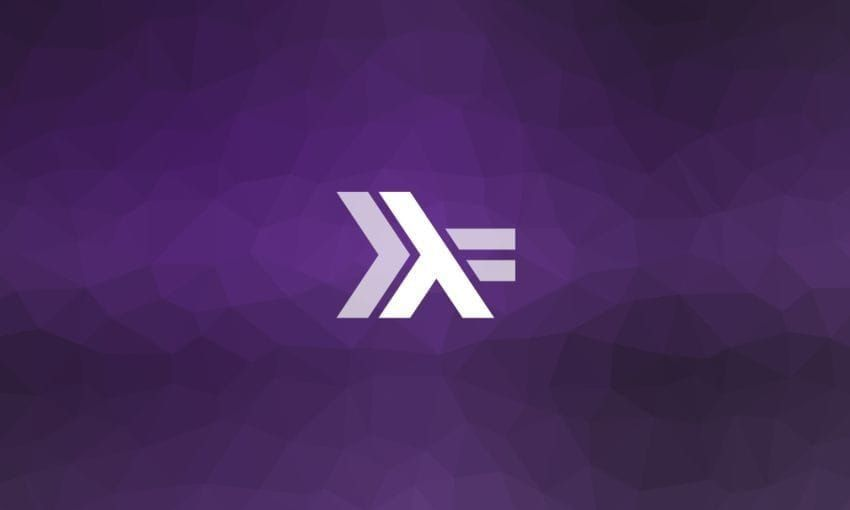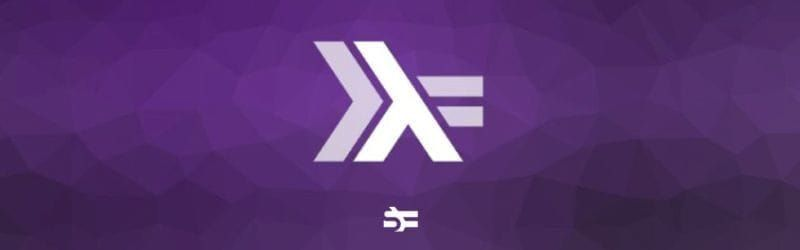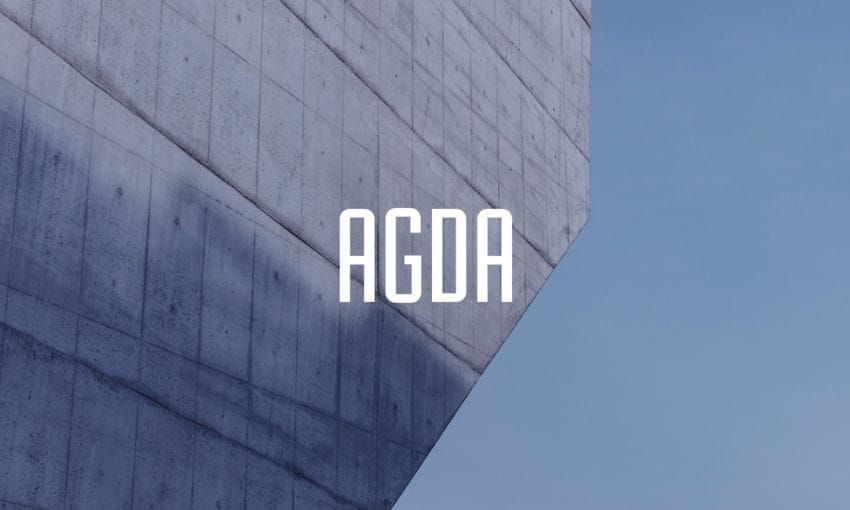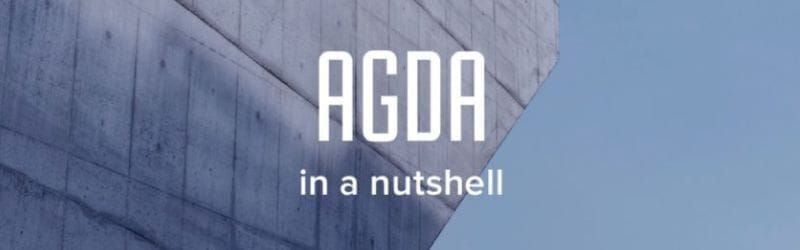# Formal Verification: History and Methods

In this series, we’ll discuss methods in formal verification. By formal verification, we mean techniques that allow one to prove statements formally and the soundness of such a proof is somehow guaranteed. This topic is full of technical aspects and different directions, our series is merely an overview. We start from the history and the roots of formal verification in mathematical logic and theoretical computer science. Such a preamble allows the readers to navigate themselves through the broad landscape of formal methods.

## A not-so-comprehensive history of formal reasoning

Historically, the idea of formal methods arose in the Modern age. The original idea of formal reasoning belongs to Gottfried Wilhelm Leibniz, a 17th-century German mathematician and philosopher. Independently from Sir Isaac Newton, he invented calculus. Moreover, Leibniz introduced the notation system for integration that we are all familiar with. The reader may take a look at the blog post written by Stephen Wolfram to explore Leibniz’s notation in more detail. By the way, Leibniz also provided the notion of a monad as a metaphysical concept. Although, such a monad has no connections with a monoid in the category of endofunctors or the same-titled type class in Haskell.

First of all, Leibniz assumed that any intellectual discourse should be described uniformly using a symbolic language similar to the algebraic notation system. This language is a tool that allows everyone to express their mathematical and philosophical thoughts. The reasoning process is supposed to be performed with certain rules according to which the soundness is guaranteed. The reasoning should be represented via calculus ratiocinator, a generalised calculus, the language of which is characteristica universalis. It was quite an ambitious idea to develop a common language of science and human knowledge. In Leibniz’s view, intellectual debates might be reduced to computation that looks like an inference in logical calculi.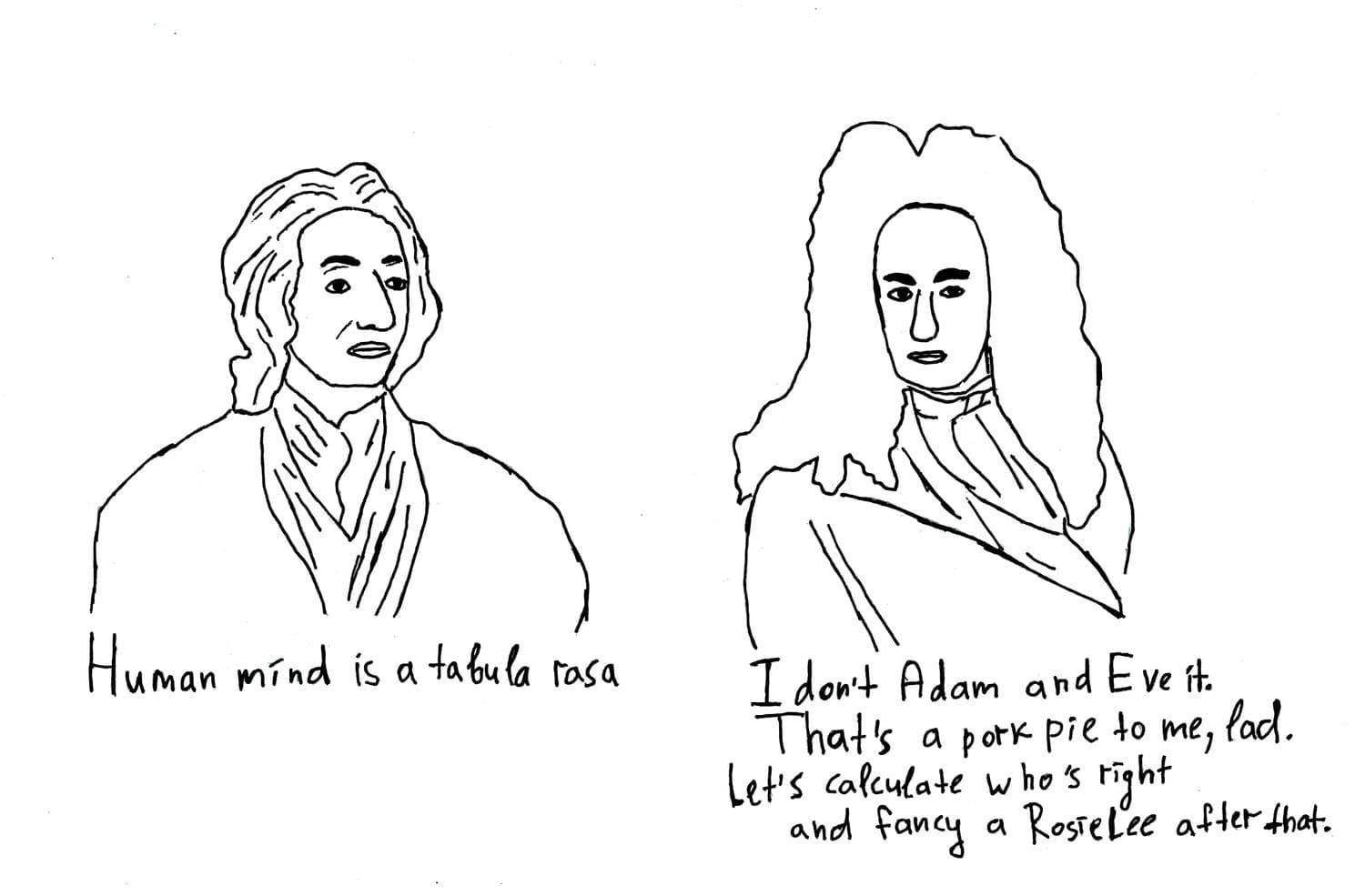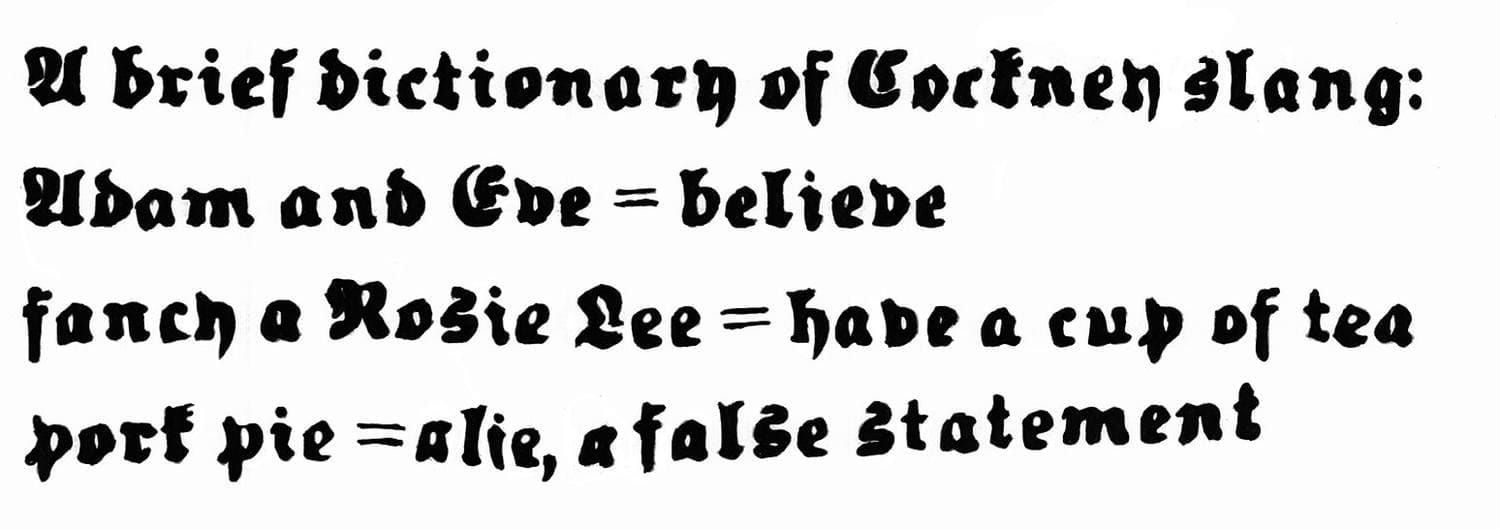In the middle of the 19th century, George Boole proposed to study Aristotelian logic algebraically. Boole, together with Augustus de Morgan, observed the correspondence between the laws of logic and the laws of the operations on sets. Those laws are known nowadays as axioms of a Boolean algebra, an abstraction of the operations on collection of elements. Now the notion of a Boolean algebra went far beyond the purely logical discourse. The algebraic consideration of reasoning via Boolean algebras became widespread after the works by Ernst Schroeder in the 1880s. In fact, those investigations launched the research in algebraic logic, one of the chronologically first branches of mathematical logic.

Alternatively, Gottlob Frege developed a framework based on syntax rather than algebraic computation. Frege’s approach is predominantly syntactic and based on a language that describes predicates and operations. This system is called Begriffsschrift, German for concept notation. Begriffsschrift is historically the first axiomatic representation of predicate logic, the logic of relations and properties with universal and existential quantifiers.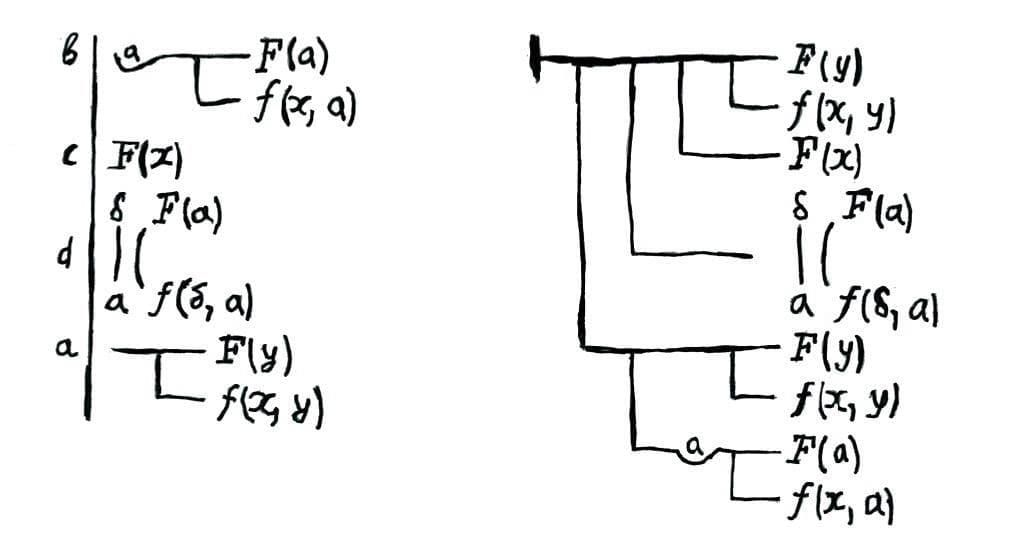An axiomatic system is a set of well-formed formulas that contains axioms and is closed under inference rules. Axioms are primitive sentences. Inference rules are a method of obtaining new theorems from existing ones. The fundamental example of an inference rule is Modus Ponens: from $A \to B$ and $A$ infer $B$. Frege’s intention was the purely logical interpretation of the underlying mathematical notions and, thus, the consideration of mathematics as a branch of logic. This programme is now called logicism.

In the 1900-s, Bertrand Russell, an English philosopher and mathematician, noted that Frege’s system is inconsistent and discovered a paradox there. Russell described the obtained paradox in his letter to Frege.

A barber is a person who shaves all those who don’t shave themselves. Does the barber shave himself?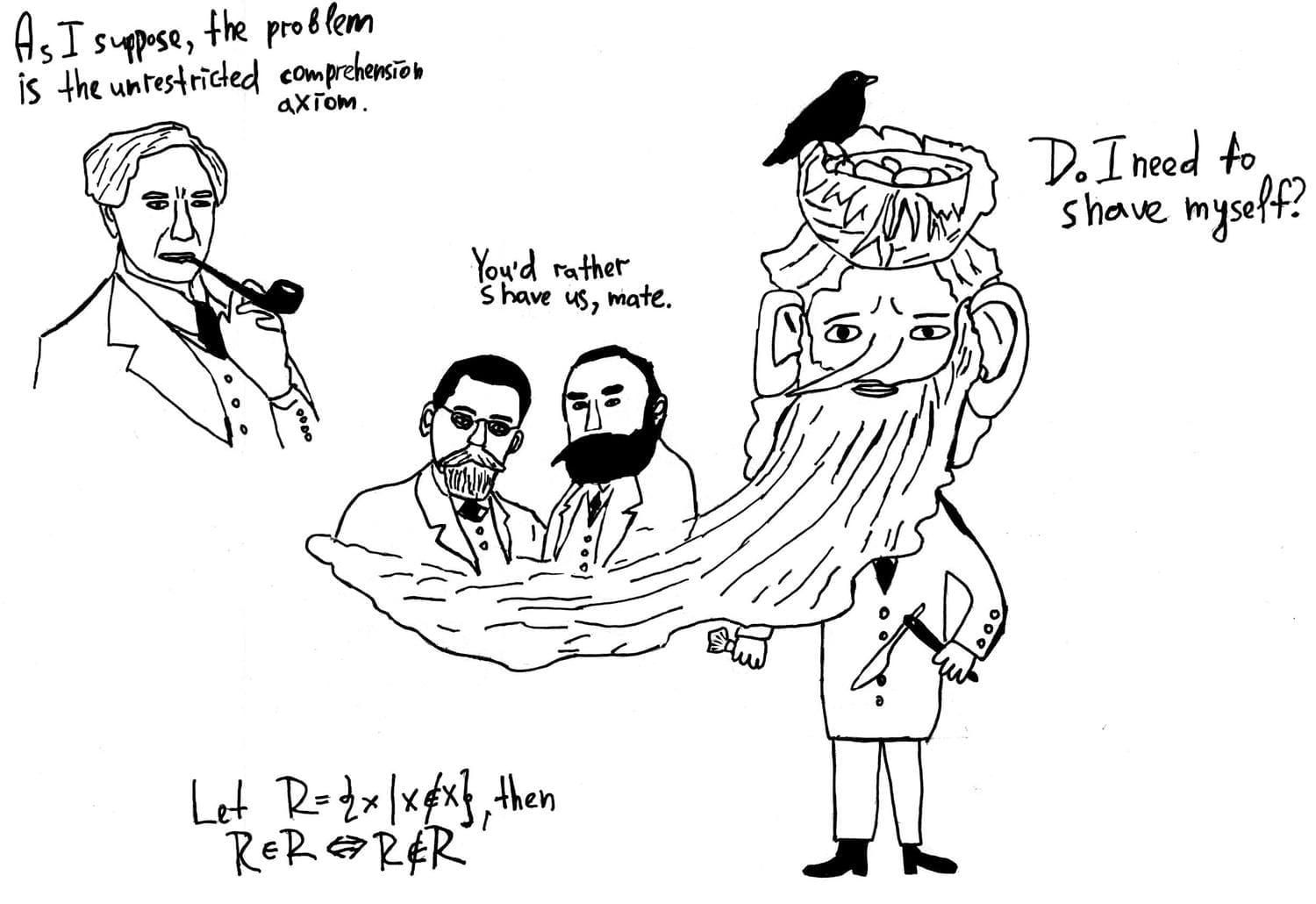Russell was a logicist in his views on the philosophy and foundations of mathematics like Frege. The aim of Russell’s programme is to describe the foundations of mathematics in a purely logical way and consistently at the same time. The result of this programme was a three-volume work called Principia Mathematica written with Alfred North Whitehead in the 1910s. The purpose of their research was an investigation of the mathematical logic language expressive capabilities in the description of the arithmetic notions. Principia Mathematica contains the very first versions of type theory proposed by Russell as an alternative to Cantor’s set theory. On the other hand, such an approach induces a completely unreadable representation of mathematical reasoning. Here’s the Principia Mathematica proof that $1 + 1 = 2$: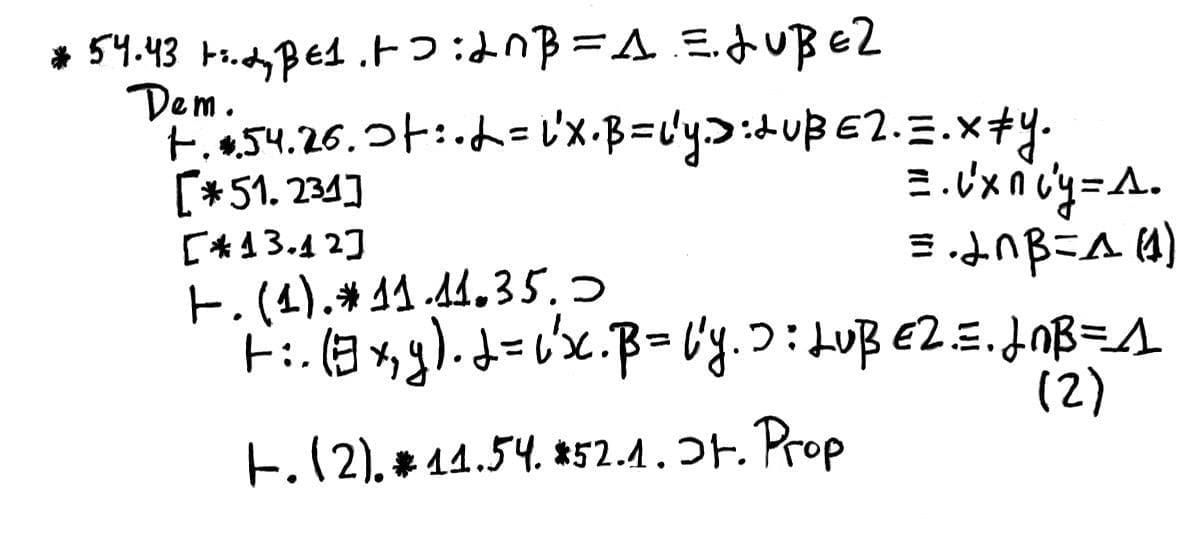We recommend watching the BBC series called The Great Philosophers, in particular, the conversation with Alfred Jules Ayer, a famous British philosopher, on the contribution of Frege and Russell to modern logic.

Now, we discuss Hilbert’s programme and the axiomatic method. We’ll take a look only at the main idea in a couple of sentences. According to this programme, any mathematical theory should have a formal axiomatic counterpart. The first attempt to represent mathematical theory axiomatically was the version of Euclidean geometry proposed by Hilbert in the 1890s. Moreover, usual mathematical proofs must be formalisable in those axiomatic theories. Hilbert also assumed that one needs to analyse derivations in those theories using finite methods that avoid, for example, the actual infinity usage. In particular, the consistency of formal axiomatic theories must be established via those finite methods.

In their turn, all those finite methods should be formalisable in Peano arithmetic, the axiomatic theory of natural numbers that, very roughly, represents mathematics of finite objects encoding them as sequences of natural numbers. Within Hilbert’s programme, such disciplines as proof theory arose that study provability, expressive capabilities, provable consistency, incompleteness, etc. Moreover, the term ‘proof theory’ belongs to Hilbert himself, Beweistheorie in origin.

As it’s well known, this programme became unrealisable when Kurt Gödel presented his restrictive theorems in 1931. The first incompleteness theorem claims that there exists a sentence of the theory of natural numbers unprovable in Peano arithmetic. Moreover, most of the theories, the language of which contains the arithmetical one, are not capable of proving their consistency within themselves. We also note that there exist examples of quite weak fragments of set theory and formal arithmetic for which the second Gödel’s theorem doesn’t hold. For example, the reader may take a look at the paper written by the author’s colleague Fedor Pakhomov of Steklov Mathematical Institute called A weak set theory that proves its own consistency.

The aspects of incompleteness and (un)provability stimulated deeper research of axiomatic theories in proof theory. Let us discuss briefly some of the directions in contemporary proof theory.

### Provably recursive functions

Computability and provability are strictly connected with each other. We recall that a function from natural numbers to natural numbers (perhaps, a many-argument one) is called computable if there exists an algorithm that computes its output for a given input. To define the notion of an algorithm strictly, one may take into consideration any existing models of computation, such as lambda calculus, Turing machines, or recursive functions.

A computable function $f$ is provably recursive in the arithmetic theory $T$ if and only if $T$ proves the formula that expresses totality of $f$: for each $x$ there exists $y$ such that $f(x) = y$. Informally, one may define an output by every input provably in $T$. Here, by an arithmetic theory, we mean a certain set of first-order formulas in the arithmetic language that consists of the $0$, $+$, $\cdot$, $\operatorname{S}$, $=$ signs. One may read $\operatorname{S}$ as a unary function symbol, an increment. That is, $\operatorname{S}(x)$ denotes x++ by means of the C++ language. Such a set of formulas also contains the axioms describing increment, addition, product, and version of the induction scheme.

The study of the provably recursive functions provides an alternative (slightly weaker) version of the first Gödel’s incompleteness theorem. Let us consider the arithmetic theory called $\operatorname{I} \Sigma_1$, a fragment of Peano arithmetic. We are not going to characterise this theory explicitly. We merely claim that the class of $\operatorname{I} \Sigma_1$-provably recursive functions is exactly primitive recursive ones that was shown by Parsons, Takeuti, and Mints in the 1960-1970s.

In the 1930-s, there was an open question: whether the class of all primitive recursive functions covers the whole class of total computable functions or not. This problem was solved negatively by Wilhelm Ackermann who provided the example of a total computable function which is not primitive recursive at all. Thus, $\operatorname{I} \Sigma_1$ cannot prove the fact that the Ackermann function is total since this function is not a primitive recursive one, as it’s well known from basic computability theory.

The standard model of $\operatorname{I} \Sigma_1$ is the set of natural numbers $\omega = \{0, 1, 2, \dots \}$ with ordinary operations on them. It is not so hard to see that the totality of the Ackermann function is true in the standard model as a sentence about natural numbers. But this statement isn’t provable in $\operatorname{I} \Sigma_1$. Thus, $\operatorname{I} \Sigma_1$ is not complete since one has the statement which is true and unprovable at the same time.

The alternative formulation of Gödel’s theorems for arithmetic theories is not the only advantage of the study of the provably recursive functions. Here, computable functions are supplied with formally provable information about their behaviour. Moreover, a characterisation of provably recursive functions provides a parameter that determines a sort of the proof-theoretical strength of a given theory. One may compare arithmetic theories by their classes of provably recursive functions. Note that there is no theory, of which the class of provably recursive functions is the whole class of total recursive functions. The reason is quite simple. When we consider the arithmetic theory, we require that the set of theorems should be enumerable. In other words, there exists an algorithm that enumerates all provable statements. On the other hand, the set of all total recursive functions is not enumerable at all. That might be shown via the Cantor’s diagonalisation procedure somewhat similar to the uncountability of the $[0,1]$ closed interval of real numbers.

If the reader is frustrated about this circumstance, we may console them. There exists a theory that proves the totality of any total recursive function. Such a theory consists of the set of all well-formed arithmetic formulas and is called trivial or degenerated. Nevertheless, we don’t advise putting this theory into practice despite the temptation to prove any formula you wish. You may accidentally prove the deliberately false statement such as $0 = 1$. We sincerely hope that the reader agrees with the author that the statement $0 = 1$ is false.

The reader may continue a more systematic study of provably recursive functions using a textbook called Proof and computation written by Helmut Schwichtenberg and Stanley S. Wainer.

### Reverse mathematics

In proof theory, we often investigate axiomatic theories and provability within them. The converse direction is to a sort of theory mining to have a minimal formal system that proves a given mathematical statement such as the Bolzano-Weierstrass theorem in real analysis. In order words, we seek the smallest set of axioms that proves the desired theorem. In reverse mathematics, one often studies subsystems of second-order arithmetic that admit quantification over sets of natural numbers. For example, this stronger quantification allows one to encode such infinite objects as real numbers representing them as Cauchy sequences of rationals.

This direction is mostly developed by logicians Harvey Friedman and Stephen Simpson. Reverse mathematics is also strongly connected with the recursion theory and constructive analysis. Such an investigation of subsystems of second-order arithmetic provides formal proofs of miscellaneous famous statements as the Brouwer fixed-point theorem and the Jordan curve theorem. The masterpiece handbook on reverse mathematics is a textbook written by Stephen Simpson called Subsystems of Second-Order Arithmetic.

### Constructive mathematics and type theory

Type theory also arises from Bertrand Russell’s ideas within the Principia Mathematica logicist programme. The initial system with types was provided as an alternative formalism to Cantor’s set theory in the works The Principles of Mathematics and Mathematical Logic as Based on the Theory of Types written during the reign of Edward VII and George V, the post-Victorian era of the British Empire.

The concept of typing was proposed to avoid such phenomenon as self-reference that implies such paradoxes as Russell’s one. A self-reference in naive set theory lies in the membership predicate $\in$ that has no restrictions at all. More precisely, the root of self-reference is the unrestricted comprehension axiom that allows one to generate sets by arbitrary predicates without any limitations. In particular, one may build the set by the predicate $\neg (x \in x)$ that yields the paradox as well. Russell’s hierarchy provides a solution for paradoxes distinguishing universes as follows. One has a zero floor of atomic elements. Any primitive element can belong only to some collection of atomic elements. In its turn, the ensemble of all collections that contain only atomic elements forms the first floor and et cetera. Moreover, this hierarchy is cumulative. That is, an element from $i$-th floor belongs to $i + 1$-th floor, etc.

The full type system was described in Principia Mathematica. This formalism full of counterintuitive axioms was too sophisticated and tangled for working mathematicians. Zermelo-Fraenkel theory, a paradox-free axiomatic version of Cantor’s set theory, arose at the same time and became much more popular than Russell’s theory of types despite the certain simplifications and enhancements proposed by Frank Plumpton Ramsey and Ludwig Wittgenstein in the 1910-1920s.

Nowadays, Zermelo-Fraenkel set theory with the axiom of choice is still the underlying foundations of mathematics by default. It is supposed that any informal mathematical construction might be reformulated strictly by means of ZFC. However, such an assumption is rather a belief based on empirical observations than a mathematical fact. Note that the Principia Mathematica system and ZFC are also incomplete since both Goedel theorems hold for these theories.

Type theory became a branch of lambda calculus in the 1940-s when Alonzo Church extended his lambda calculus with types to avoid self-application in lambda terms. The initial variant of typed lambda calculus was a combination of Russell’s type theory with lambda calculus. Further, the connection between typed lambda calculus and constructive mathematics was discovered by Haskell Curry and William Howard. The reader may take a look at the first part of our blog post series on (non)constructive proofs in Agda, where the historical and philosophical origins of constructive mathematics are explained briefly.

Type theory as a branch of proof theory influenced programming language theory in two ways. The first perspective includes the theory of programming languages with types. The development of type systems initially evolved from a proof-theoretical perspective. For example, polymorphic lambda calculus (widely known as the system $\operatorname{F}$) was proposed by Jean-Yves Girard to characterise the class of provably recursive functions of intuitionistic second-order arithmetic. Nowadays, we consider the system $\operatorname{F}$ as an axiomatic description of parametric polymorphism implemented in Haskell.

The alternative perspective has its origins in the foundations of constructive mathematics. This direction was developed by Per Martin-Löf in the 1970s. The idea was to provide a logical symbolic system that was adequate as a foundation of constructive mathematics and a system of typed lambda calculus at the same time. That is, such a formalism should answer philosophical questions on the underlying mathematical notions while being a programming language. Nowadays, this system is called constructive type theory. From a programmer’s perspective, this formalism provides systems of dependently typed lambda calculus. Thus, type systems propose underlying formalisms for the programming languages that do Curry-Howard based formal verification. We’ll discuss some prospective dependently typed languages such as F*, Lean, and Arend later.

## Conclusions

We have taken a look at the history and mathematical origins and aspects of formal verification. Our essay doesn’t cover the whole historical development indeed, but we emphasised several turning episodes that influenced formal methods as we know them. We surveyed such directions of proof theory as provably recursive functions, reverse mathematics, type theory within constructive mathematics. These directions are not the only ones. We dropped such branches as ordinal analysis and proof mining, each of which also merits a different post series. In the second part, we will discuss the practical aspects of the disciplines we considered here. In particular, we will introduce the reader to SMT solvers, program logics, model checking, and dependently typed languages. We believe that our series will provide you the bridge between theoretical foundations and practical issues.

Formal Verification: History and MethodsMore from Serokell